利用划分子集限制连接池的大小(1)

1. 引言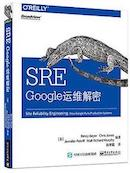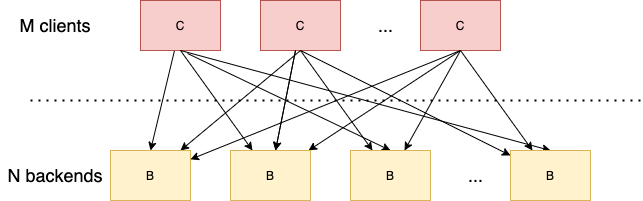2. 子集选择算法带来的思考

2.1 目标

1) Backend的负载是否均衡
2) 当Backend集群发生变化(比如有实例宕机，或者进行滚动发布或者重启时，Client的连接池是否有大量的连接需要创建或者销毁
3) 当Backend集群发生变化(比如有实例宕机，或者进行滚动发布或者重启时，Client的请求时的错误率相比没有划分子集时，是否会变大

2.2 子集选择算法

2.2.1 随机选择

clientSize=50
backendSize=100
subsetSize=50

library(ggplot2)
ggplot(data, aes(X, Y)) + geom_bar(stat = 'identity')

subset/random/random.go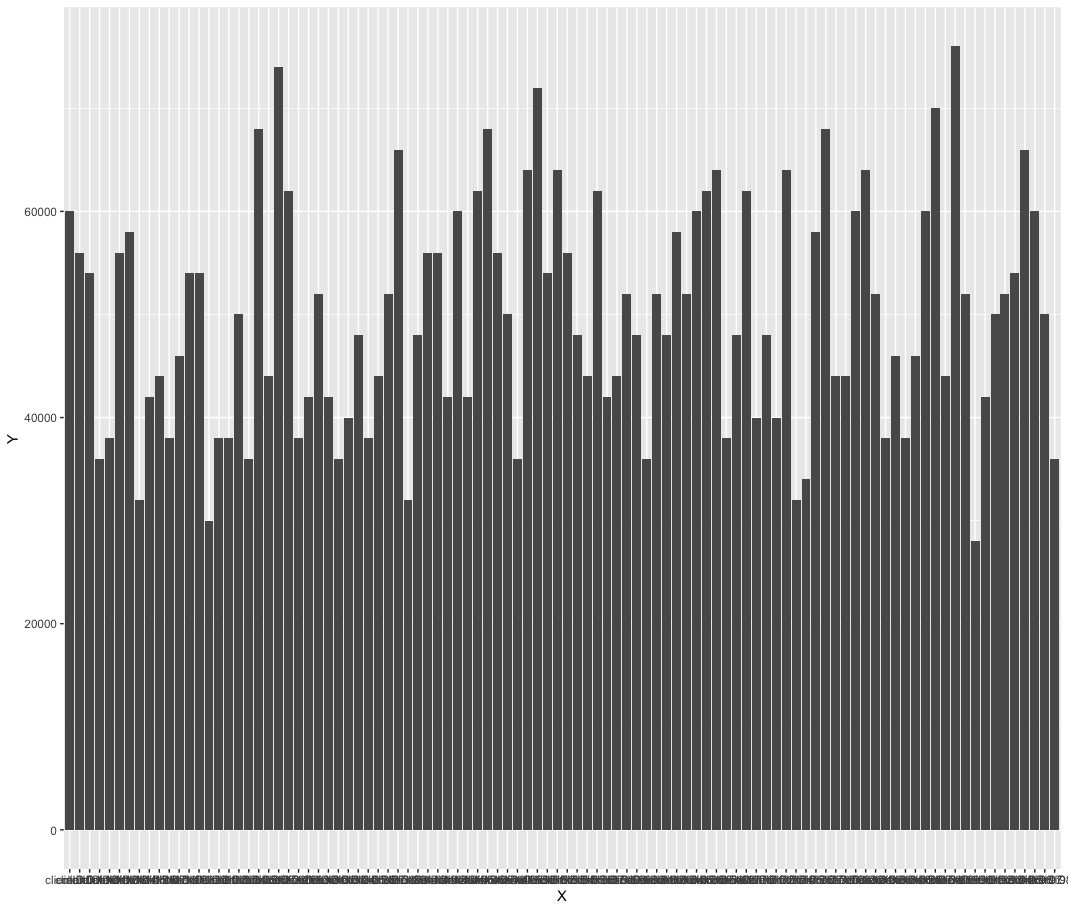2.2.2 确定性算法

func Subset(backends []string, clientID, subsetSize int) []string {

subsetCount := len(backends) / subsetSize

// Group clients into rounds; each round uses the same shuffled list:
round := clientID / subsetCount

r := rand.New(rand.NewSource(int64(round)))
r.Shuffle(len(backends), func(i, j int) { backends[i], backends[j] = backends[j], backends[i] })

// The subset id corresponding to the current client:
subsetID := clientID % subsetCount

start := subsetID * subsetSize
return backends[start : start+subsetSize]
}

subset/certainty1/verify.go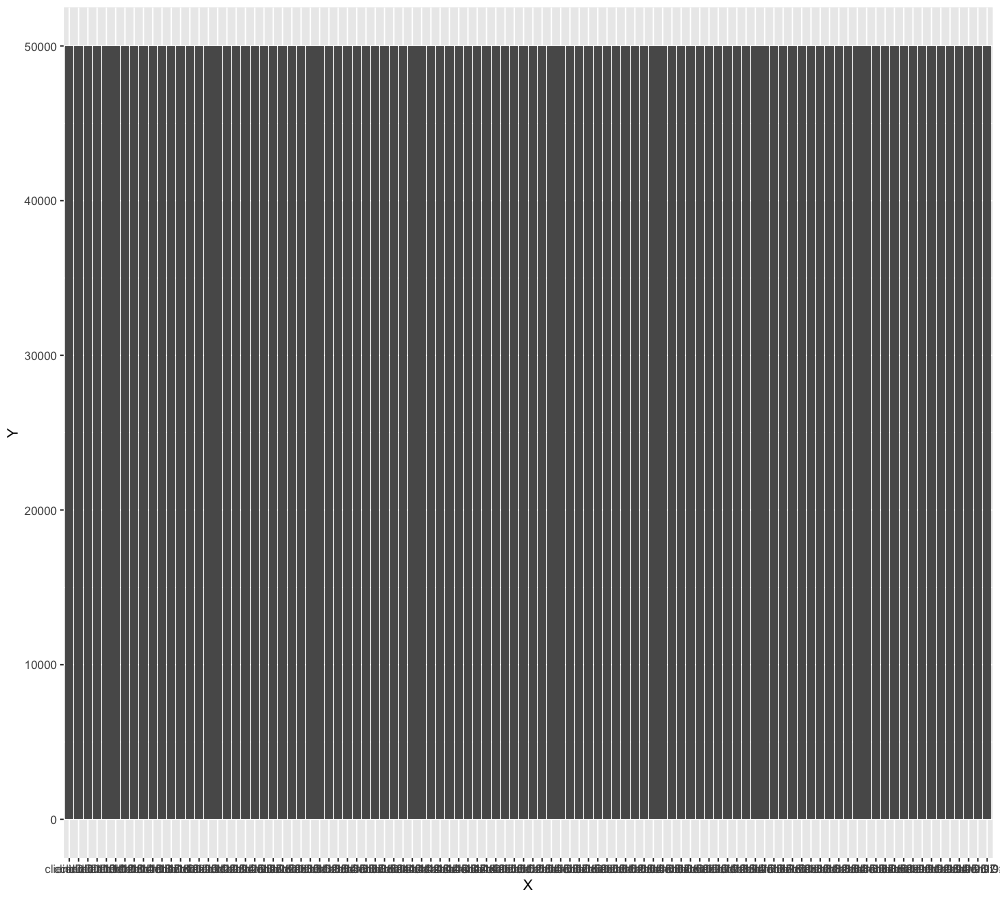• clientID必须连续
• backendSize要是subsetSize的整数倍，或者接近整数倍

反例1

subset/certainty2/verify.go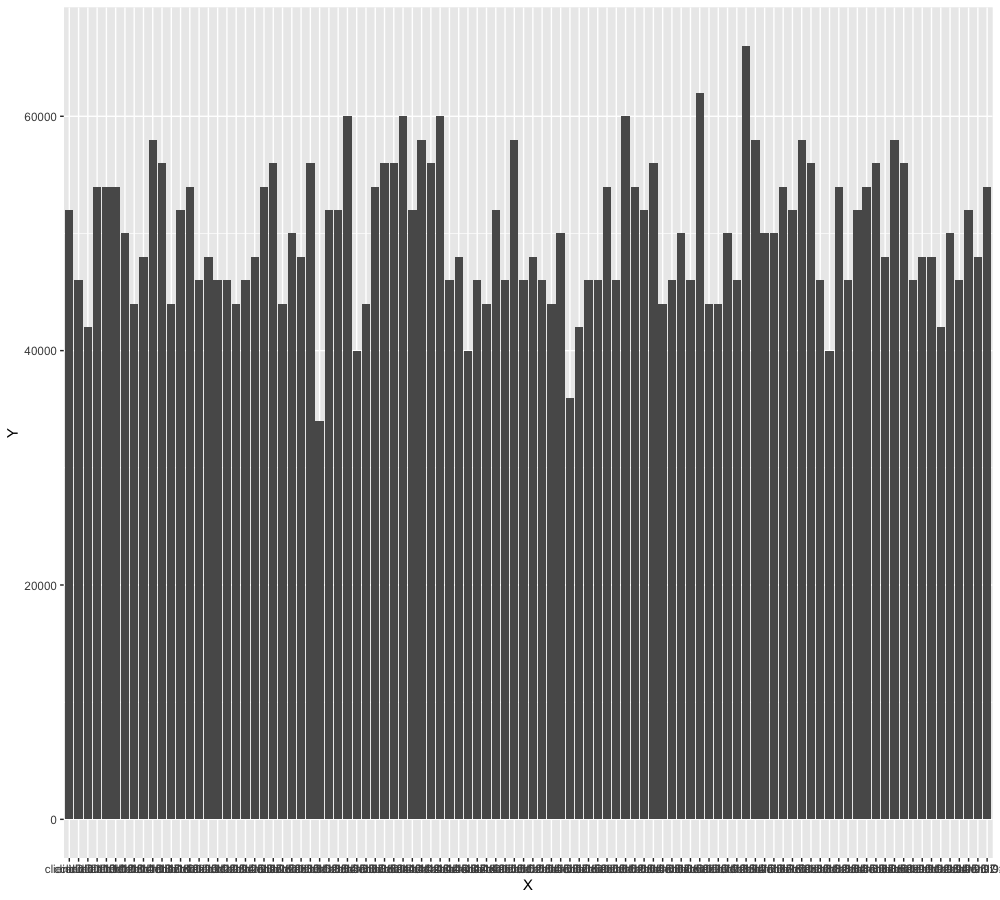反例2

subset/certainty3/verify.go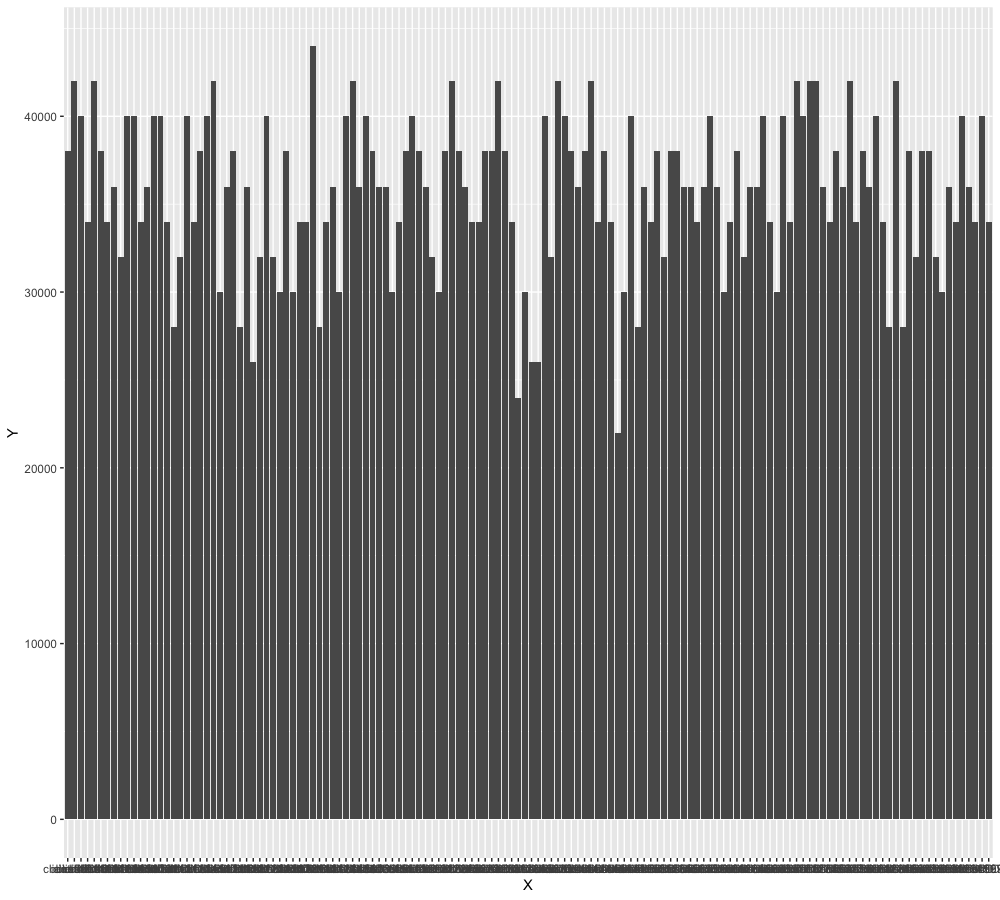参考资料

打赏我1. 匿名说道：

赞，好文